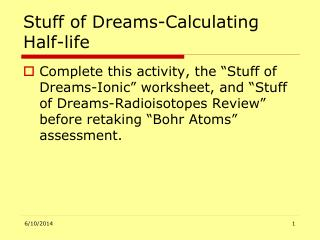DownloadDownload PresentationStuff of Dreams-Calculating Half-life

# Stuff of Dreams-Calculating Half-life

Télécharger la présentation## Stuff of Dreams-Calculating Half-life

- - - - - - - - - - - - - - - - - - - - - - - - - - - E N D - - - - - - - - - - - - - - - - - - - - - - - - - - -
##### Presentation Transcript

1. Stuff of Dreams-Calculating Half-life • Complete this activity, the “Stuff of Dreams-Ionic” worksheet, and “Stuff of Dreams-Radioisotopes Review” before retaking “Bohr Atoms” assessment.

2. The problem-evaluate • The half-life of iodine-130 is 20 days. A tube containing 100 picograms of iodine-130 is stored in a vial. How many picograms are left after 40 days? • Evaluate the problem—4 numbers are given: two with time (days) units, one with mass in a grams unit (picograms) and another mass given as mass number with iodine-130. Always ignore the mass number-it wont help solve for half-life

3. The problem-solve • The half-life of iodine-130 is 20 days. A tube containing 100 picograms of iodine-130 is stored in a vial. How many picograms are left after 40 days? • Solve the problem—use the two numbers with time units two calculate the number of half-lifes the element has gone through. Do this by dividing the largest time unit by the smallest time unit. • 40 days = 2 half-lives 20 days

4. Iodine—130 • The half-life of iodine-130 is 20 days. A tube containing 100 picograms of iodine-130 is stored in a vial. How many picograms are left after 40 days? • Solve the problem—the number of half-lives previously calculated tells you how many timesto divide the amount of isotope (100 picograms of iodine-130) in half—2! • 100 picograms = 50 picograms 2 3. 50 picograms = 25 picograms YOUR ANSWER 2 Draw a box chart to illustrate 50 Pico grams 100 Picograms Iodine-130 25 Pico grams

5. Carbon—14 The half-life of carbon-14 is approximately 5000 years. A tube containing 200 micrograms of carbon-14 is stored in a vial. How many micrograms are left after 5000 years? • 5000 years = 1 half life 5000 years 2. 200 picograms = 100 micrograms Your answer! 2 Draw a box chart to illustrate 100 micro grams 200 micrograms Carbon-14

6. Strontium—87 • The half-life of strontium-87 is approximately 15 minutes. A tube containing 88 grams of strontium-87 is stored in a vial. How many grams are left after 45 minutes? 1. 45 minutes = 3 half lives 15 minutes 2. 88 grams = 44 grams 2 44 grams = 22 grams 2 22 grams = 11 grams 2 • Draw a box chart to illustrate 44 grams 22 grams 11 grams 88 gram Strontium-87

7. Hafnium—75 • The half-life of hafnium-75 is approximately 24 milliseconds. A tube containing 4 micrograms of hafnium-75 is stored in a vial. How many milligrams are left after 48 milliseconds?

8. Calcium—46 • The half-life of calcium-46 is 26 years. A tube containing 18 milligrams of calcium-46 is stored in a vial. How many micrograms are left after 26 years?